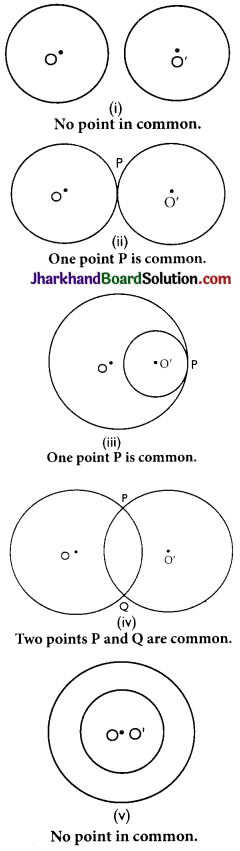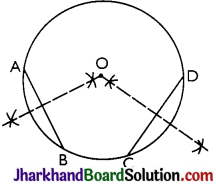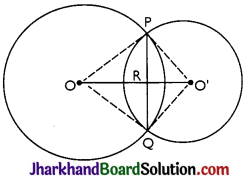# JAC Class 9 Maths Solutions Chapter 10 Circles Ex 10.3

Jharkhand Board JAC Class 9 Maths Solutions Chapter 10 Circles Ex 10.3 Textbook Exercise Questions and Answers.

## JAC Board Class 9th Maths Solutions Chapter 10 Circles Ex 10.3

Page-176

Question 1.
Draw different pairs of circles. How many points does each pair have in common? What is the maximum number of common points?So, maximum number of common points = 2

Question 2.
Suppose you are given a circle. Give a construction to find its centre.Steps of construction:
Step I: A circle is drawn.
Step II: Two chords AB and CD are drawn.
Step III: Perpendicular bisector of the chords AB and CD are drawn.
Step IV: Let these two perpendicular bisectors meet at a point. The point of intersection of these two perpendicular bisectors is the centre of the circle.Question 3.
If two circles intersect at two points, prove that their centres lie on the perpendicular bisector of the common chord.Given: Two circles which intersect each other at P and Q.
To prove: 00′ is perpendicular bisector of PQ.
Proof: In ∆POO’ and ∆QOO’,
OO’ = 00′ (Common)
∆POO’ ≅ ∆QOO’
(SSS congruence criterion)
Thus,
∠POO’ = ∠QOO’ (CPCT) …(i)
In ∆POR and ∆QOR,
⇒ ∠PRO = ∠QRO = $$\frac{180°}{2}$$ = 90°